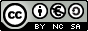Primary Education INDEX Introduction Objectives 1 - The square metre, multiples and submutiples of the square metre. 2 - Area unit conversion 3 - Area unit conversion. 4 - Area units for fields 5 - Match the equal measurements. 6 - Find the area. 1 - The cubic meter, multiples and submultiples of the cubic metre. 2 - Volume unit conversion. 3 - Volume unit conversion. 4 - Match the equal volumes. 5 - Find the volume. 1 -  International System (SI) base units.
Square metres, cubic metres
INTRODUCTION

These activities are designed to be viewed on a 17-inch screen and with a resolution of 1024 x 768 pixels because some windows show measurements in centimetres and millimetres that, when viewed with another configuration, won't correspond with reality; and the size of some windows can even be too big.

This unit only deals with a basic knowledge on length, volume and weight units, as studied in primary school. It does not deal with the other units of the International System.

Moreover, the student should perform measurements and volume or area calculations in order to learn and get used to handling measurement units. This cannot be done on the computer.

OBJECTIVES
• To learn the concepts of square metre and cubic metre, its multiples and submultiples.
• To reinforce confidence and skills in surface and volume unit conversion.
• To practise the measurement and the rectangle area calculation, and to express it in  square centimetres and square millimetres.
• To practise the measurement and the cuboid (rectangular prism) area calculation, and to express it in square centimetres and square millimetres.
• To get an accurate measurement and calculation and the accurate expression of the values.
 Eduardo Barbero CorralSpanish Ministry of Education. Year 2007